## Pandas, Numpy, JSON & SSL (Crossover of Space Stone & Reality Stone in Python Verse)

In our last installment, we’ve shown pandas & numpy based on a specific situation. If that is our Space Stone installment of Python Verse, then this would be one approach of creating much interesting crossover of Space Stone & Reality Stone of Python verse. Yes. You are right. We’ll be discussing one requirement, where we need many of these in a single task.

Let’s dive into it!

Let’s assume that we have a source csv file which has the following data –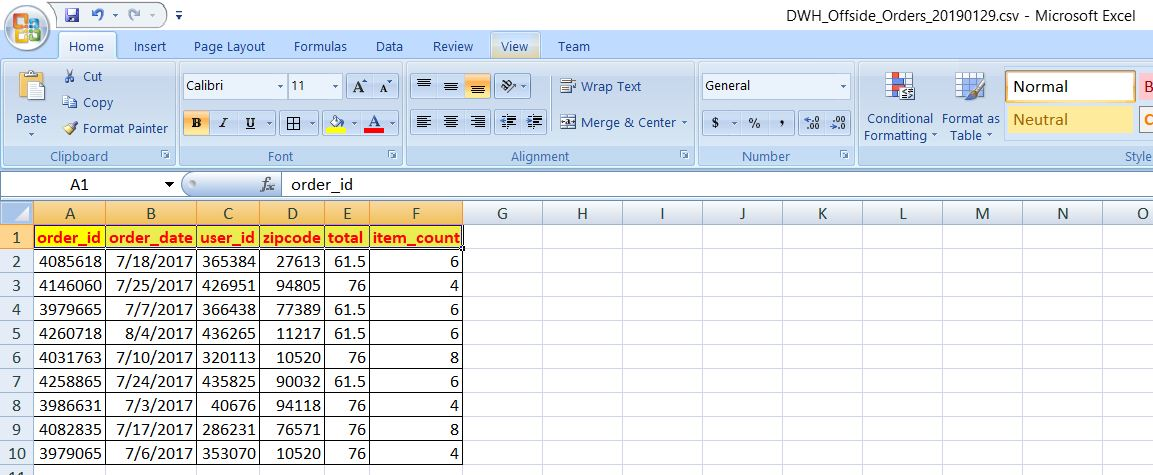Now, the requirement is – we need to use one third party web service to send JSON payload preparing with this data & send them to the 3rd party API to get the City, State & based on that we need to find the total number of item sold against each State & City.Let’s look into our third-party API site  –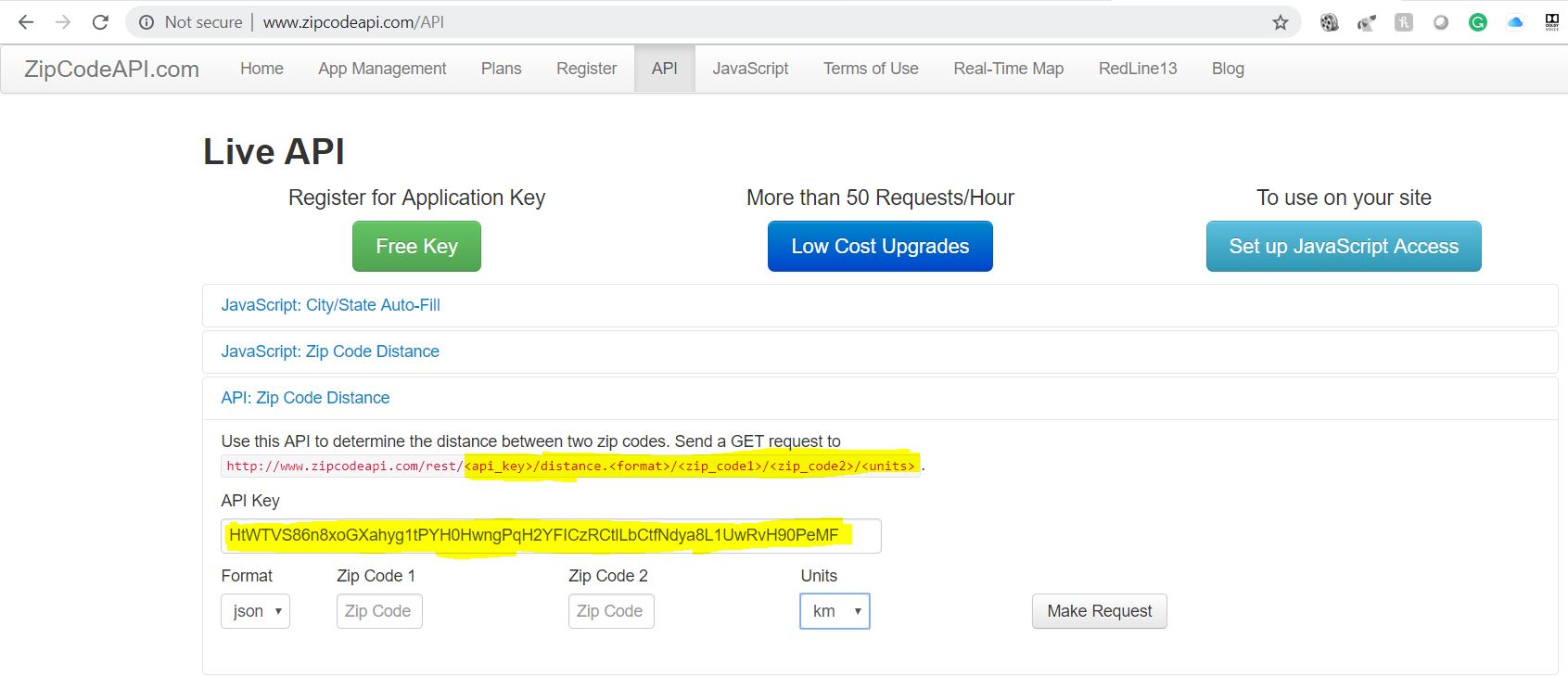As per the agreement with this website, any developer can test 10 calls per day free. After that, it will send your response with encrypted values, e.g. Classified. But, we don’t need more than 10 calls to test it.

Here, we’ll be dealing with the 4 python scripts. Among them, one scenario I’ve already described in my previous post. So, I’ll be just mentioning the file & post the script.

Please find the directory structure in both the OS –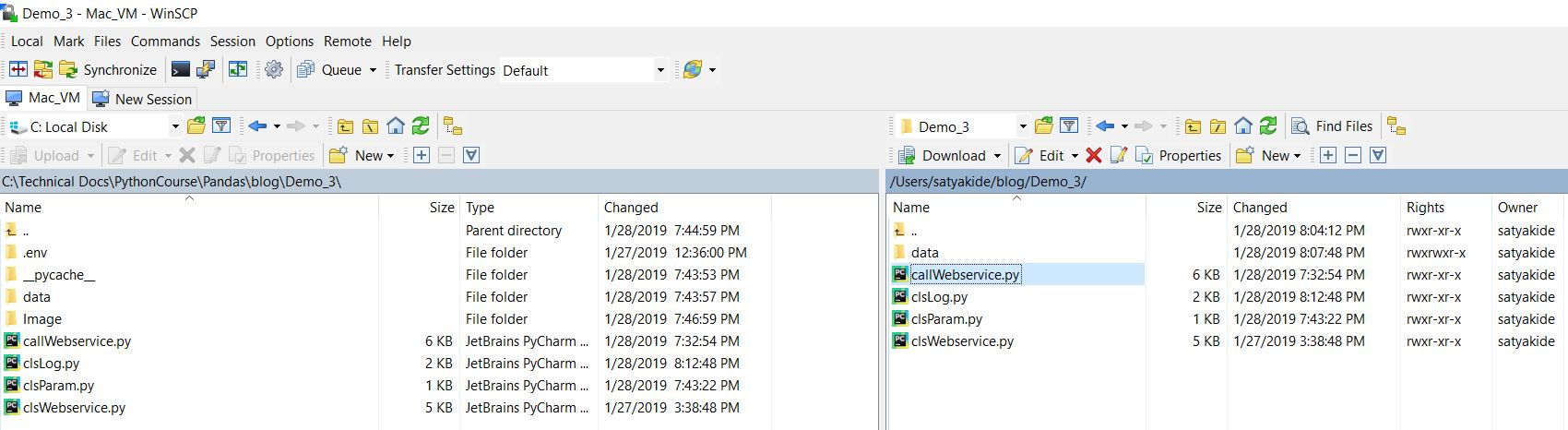1. clsLpy (This script will create the split csv files or final merge file after the corresponding process. However, this can be used as usual verbose debug logging as well. Hence, the name comes into the picture.)

 ``` 1 2 3 4 5 6 7 8 9 10 11 12 13 14 15 16 17 18 19 20 21 22 23 24 25 26 27 28 29 30 31 32 33 34 35 36 37 38 39 40 41``` ```########################################### #### Written By: SATYAKI DE ######## #### Written On: 20-Jan-2019 ######## ########################################### import pandas as p import os import platform as pl from clsParam import clsParam as cf class clsL(object): def __init__(self): self.path = cf.config['PATH'] def logr(self, Filename, Ind, df, subdir=None): try: x = p.DataFrame() x = df sd = subdir os_det = pl.system() if sd == None: if os_det == "Windows": fullFileName = self.path + '\\' + Filename else: fullFileName = self.path + '/' + Filename else: if os_det == "Windows": fullFileName = self.path + '\\' + sd + "\\" + Filename else: fullFileName = self.path + '/' + sd + "/" + Filename if Ind == 'Y': x.to_csv(fullFileName, index=False) return 0 except Exception as e: y = str(e) print(y) return 3 ```

2. clsParam.py (This script contains the parameter entries in the form of dictionary & later this can be used in all the relevant python scripts as configuration parameters.)

 ``` 1 2 3 4 5 6 7 8 9 10 11 12 13 14 15``` ```########################################### #### Written By: SATYAKI DE ######## #### Written On: 20-Jan-2019 ######## ########################################### import os class clsParam(object): config = { 'MAX_RETRY' : 5, 'API_KEY' : 'HtWTVS86n8xoGXahyg1tPYH0HwngPqH2YFICzRCtlLbCtfNdya8L1UwRvH90PeMF', 'PATH' : os.path.dirname(os.path.realpath(__file__)), 'SUBDIR' : 'data' } ```

As you can see from this script that we’ve declared all the necessary parameters here as dictionary object & later we’ll be referring these parameters in the corresponding python scripts.

`'API_KEY' : 'HtWTVS86n8xoGXahyg1tPYH0HwngPqH2YFICzRCtlLbCtfNdya8L1UwRvH90PeMF'`

One crucial line, we’ll look into. API_KEY will be used while sending the JSON payload to the third-party web service. We’ll get this API_KEY from the highlighted (In Yellow) picture posted above.

3. clsWeb.py (This is the main script, which will first convert the pandas’ data frames into JSON & and send the API request as per the third party site.  It will capture the response & convert that by normalizing the data & poured it back to the data frame for further process.)

 ``` 1 2 3 4 5 6 7 8 9 10 11 12 13 14 15 16 17 18 19 20 21 22 23 24 25 26 27 28 29 30 31 32 33 34 35 36 37 38 39 40 41 42 43 44 45 46 47 48 49 50 51 52 53 54 55 56 57 58 59 60 61 62 63 64 65 66 67 68 69 70 71 72 73 74 75 76 77 78 79 80 81 82 83 84 85 86 87 88 89 90 91 92 93 94 95 96 97 98 99 100 101 102 103 104 105 106 107 108 109 110 111 112 113 114 115 116 117 118 119 120 121 122 123 124 125 126 127 128 129 130 131 132 133 134 135 136 137 138 139 140 141 142 143 144 145 146 147 148 149 150``` ```########################################### #### Written By: SATYAKI DE ######## #### Written On: 20-Jan-2019 ######## ########################################### import json import requests import datetime import time import ssl from urllib.request import urlopen import pandas as p import numpy as np import os import gc from clsParam import clsParam as cp class clsWeb(object): def __init__(self, payload, format, unit): self.payload = payload self.path = cp.config['PATH'] # To disable logging info self.max_retries = cp.config['MAX_RETRY'] self.api_key = cp.config['API_KEY'] self.unit = unit self.format =format def get_response(self): # Assigning Logging Info max_retries = self.max_retries api_key = self.api_key unit = self.unit format = self.format df_conv = p.DataFrame() cnt = 0 try: # Bypassing SSL Authentication try: _create_unverified_https_context = ssl._create_unverified_context except AttributeError: # Legacy python that doesn't verify HTTPS certificates by default pass else: # Handle target environment that doesn't support HTTPS verification ssl._create_default_https_context = _create_unverified_https_context # Capturing the payload data_df = self.payload temp_df = data_df[['zipcode']] list_of_rec = temp_df['zipcode'].values.tolist() print(list_of_rec) for i in list_of_rec: zip = i # Providing the url url_part = 'http://www.zipcodeapi.com/rest/' url = url_part + api_key + '/' + 'info.' + format + '/' + str(zip) + '/' + unit headers = {"Content-type": "application/json"} param = headers var1 = datetime.datetime.now().strftime("%H:%M:%S") print('Json Fetch Start Time:', var1) retries = 1 success = False while not success: # Getting response from web service response = requests.get(url, params=param, verify=False) # print("Complete Error:: ", str(response.status_code)) # print("Error First::", str(response.status_code)[:1]) if str(response.status_code)[:1] == '2': # response = s.post(url, params=param, json=json_data, verify=False) success=True else: wait = retries * 2 print("Retry fails! Waiting " + str(wait) + " seconds and retrying.") time.sleep(wait) retries += 1 # Checking Maximum Retries if retries == max_retries: success=True raise ValueError # print(response.text) var2 = datetime.datetime.now().strftime("%H:%M:%S") print('Json Fetch End Time:', var2) print("-" * 90) # Capturing the response json from Web Service df_response_json = response.text string_to_json = json.loads(df_response_json) # Converting the response json to Dataframe # df_Int_Rec = p.read_json(string_to_json, orient='records') df_Int_Rec = p.io.json.json_normalize(string_to_json) df_Int_Rec.columns = df_Int_Rec.columns.map(lambda x: x.split(".")[-1]) if cnt == 0: df_conv = df_Int_Rec else: d_frames = [df_conv, df_Int_Rec] df_conv = p.concat(d_frames) cnt += 1 # Deleting temporary dataframes & Releasing memories del [[df_Int_Rec]] gc.collect() # Resetting the Index Value df_conv.reset_index(drop=True, inplace=True) # Merging two data side ways maintaining the orders df_add = p.concat([data_df, df_conv], axis=1) del [[df_conv]] gc.collect() # Dropping unwanted column df_add.drop(['acceptable_city_names'], axis=1, inplace=True) return df_add except ValueError as v: print(response.text) x = str(v) print(x) # Return Empty Dataframe df = p.DataFrame() return df except Exception as e: print(response.text) x = str(e) print(x) # Return Empty Dataframe df = p.DataFrame() return df ```

Let’s look at the key lines to discuss –

```def __init__(self, payload, format, unit):

self.path = cp.config['PATH']

# To disable logging info
self.max_retries = cp.config['MAX_RETRY']
self.api_key = cp.config['API_KEY']
self.unit = unit
self.format = format```

The first block will be instantiated as soon as you are invoking the class. Note that, we’ve used our parameter class python script here as cp & then we’re referring the corresponding elements as & when requires. Other parameters will be captured from the invoking script, which we’ll be discussed later in this post.

```# Bypassing SSL Authentication
try:
_create_unverified_https_context = ssl._create_unverified_context
except AttributeError:
# Legacy python that doesn't verify HTTPS certificates by default
pass
else:
# Handle target environment that doesn't support HTTPS verification
ssl._create_default_https_context = _create_unverified_https_context```

Sometimes, Your Firewall or Proxy might block your web service request due to a specific certificate error. This snippet will bypass that authentication. However, it is always advised to use proper SSL certification in the Production environment.

```# Capturing the payload
temp_df = data_df[['zipcode']]

list_of_rec = temp_df['zipcode'].values.tolist()```

In this snippet, we’re capturing the zip code from our source data frame & converting them into a list & this would be our candidate to pass the data as part of our JSON payload.

```for i in list_of_rec:
zip = i

# Providing the url
url_part = 'http://www.zipcodeapi.com/rest/'
url = url_part + api_key + '/' + 'info.' + format + '/' + str(zip) + '/' + unit

Once, we’ve extracted our zip codes, we’re passing it one-by-one & forming our JSON with header & data.

```retries = 1
success = False

while not success:
# Getting response from web service
response = requests.get(url, params=param, verify=False)
# print("Complete Error:: ", str(response.status_code))
# print("Error First::", str(response.status_code)[:1])

if str(response.status_code)[:1] == '2':
# response = s.post(url, params=param, json=json_data, verify=False)
success=True
else:
wait = retries * 2
print("Retry fails! Waiting " + str(wait) + " seconds and retrying.")
time.sleep(wait)
retries += 1

# Checking Maximum Retries
if retries == max_retries:
success=True
raise ValueError```

In this section, we’re posting our JSON application & waiting for the response from the third-party API. If we receive the success response (200), we will proceed with the next zip code. However, if we didn’t receive the success response, we’ll retry the post option again until or unless it reaches the maximum limits. In case, if the application still waiting for a valid answer even after the maximum limit, it will exit from the loop & raise an error to the main application.

```# Capturing the response json from Web Service
df_response_json = response.text

# Converting the response json to Dataframe
df_Int_Rec = p.io.json.json_normalize(string_to_json)
df_Int_Rec.columns = df_Int_Rec.columns.map(lambda x: x.split(".")[-1])```

This snippet will extract the desired response from the API & convert that back to the Pandas data frame. Last two lines, it is normalizing the data that it has received from the API for further process. This is critical steps as these steps will lead to extract City & State from our API response.

```# Merging two data side ways maintaining the orders

Once, we’ll have structured data – we can merge it back to our source data frame for our next step.

4. callWebservice.py (This script will call the API script & also process the data to create an aggregate report for our task.)

 ``` 1 2 3 4 5 6 7 8 9 10 11 12 13 14 15 16 17 18 19 20 21 22 23 24 25 26 27 28 29 30 31 32 33 34 35 36 37 38 39 40 41 42 43 44 45 46 47 48 49 50 51 52 53 54 55 56 57 58 59 60 61 62 63 64 65 66 67 68 69 70 71 72 73 74 75 76 77 78 79 80 81 82 83 84 85 86 87 88 89 90 91 92 93 94 95 96 97 98 99 100 101 102 103 104 105 106 107 108 109 110 111 112 113 114 115 116 117 118 119 120 121 122 123 124 125 126 127 128 129 130 131 132 133 134 135 136 137 138 139 140 141 142 143 144 145 146 147 148 149 150 151 152 153 154``` ```##################################################### ### Objective: Purpose of this Library is to call ### ### the Web Service method to capture the city, ### ### & the state as a json response & update them ### ### in the dataframe & finally produce the summary### ### of Total Sales & Item Counts based on the City### ### & the State. ### ### ### ### Arguments are as follows: ### ### Mentioned the Exception Part. First time dry ### ### run the program without providing any args. ### ### It will show all the mandatory params. ### ### ### ##################################################### ##################################################### #### Written By: SATYAKI DE ### #### Written On: 20-Jan-2019 ### ##################################################### import clsWeb as cw import sys import pandas as p import os import platform as pl import clsLog as log import datetime import numpy as np from clsParam import clsParam as cp # Disbling Warnings def warn(*args, **kwargs): pass import warnings warnings.warn = warn def main(): print("Calling the custom Package..") try: if len(sys.argv) == 4: inputFile = str(sys.argv) format = str(sys.argv) unit = str(sys.argv) else: raise Exception # Checking whether the format contains # allowable choices or not if (format == 'JSON'): format = 'json' elif (format == 'CSV'): format = 'csv' elif (format == 'XML'): format = 'xml' else: raise Exception # Checking whether the format contains # allowable choices or not if (unit == 'DEGREE'): unit = 'degree' elif (unit == 'RADIANS'): unit = 'radians' else: raise Exception print("*" * 170) print("Reading from " + str(inputFile)) print("*" * 170) # Initiating Logging Instances clog = log.clsLog() path = cp.config['PATH'] subdir = cp.config['SUBDIR'] os_det = pl.system() if os_det == "Windows": src_path = path + '\\' + 'data\\' else: src_path = path + '/' + 'data/' # Reading source data csv file df_Payload = p.read_csv(src_path+inputFile, index_col=False, skipinitialspace=True) x = cw.clsWeb(df_Payload, format, unit) retDf = x.get_response() # Total Number of rows fetched count_row = retDf.shape if count_row == 0: print("Data Processing Issue!") else: print("Writing to file -> (" + str(inputFile) + "_modified.csv) Status: Success") FileName, FileExtn = inputFile.split(".") # Writing to the file clog.logr(FileName + '_modified.' + FileExtn, 'Y', retDf, subdir) print("*" * 170) # Performing group by operation to get the desired result # State & City-wise total Sales & Item Sales df_src = p.DataFrame() df_src = retDf[['city', 'state', 'total', 'item_count']] # Converting values to Integer df_src['city_1'] = retDf['city'].astype(str) df_src['state_1'] = retDf['state'].astype(str) df_src['total_1'] = retDf['total'].astype(int) df_src['item_count_1'] = retDf['item_count'].astype(int) # Dropping the old Dtype Columns df_src.drop(['city'], axis=1, inplace=True) df_src.drop(['state'], axis=1, inplace=True) df_src.drop(['total'], axis=1, inplace=True) df_src.drop(['item_count'], axis=1, inplace=True) # Renaming the new columns to as per Old Column Name df_src.rename(columns={'city_1': 'city'}, inplace=True) df_src.rename(columns={'state_1': 'state'}, inplace=True) df_src.rename(columns={'total_1': 'total'}, inplace=True) df_src.rename(columns={'item_count_1': 'item_count'}, inplace=True) # Performing Group By Operation grouped = df_src.groupby(['state', 'city']) res_1 = grouped.aggregate(np.sum) print("DF:") print(res_1) FileName1 = 'StateCityWiseReport' # Handling Multiple source files var = datetime.datetime.now().strftime(".%H.%M.%S") print('Target File Extension will contain the following:: ', var) # Writing to the file clog.logr(FileName1 + var + '.' + FileExtn, 'Y', df_src, subdir) print("*" * 170) print("Operation done for " + str(inputFile) + "!") print("*" * 170) except Exception as e: x = str(e) print(x) print("*" * 170) print('Current order would be - <' + str(sys.argv) + '> ') print('Make sure last two params should be in CAPS only!') print("*" * 170) if __name__ == "__main__": main() ```

Let’s look at some vital code snippet in this main script –

```# Reading source data csv file

retDf = x.get_response()```

In this snippet, we’re getting our data from our source csv & then calling our leading Web API service to get the State & City information.

```# Converting values to Integer
df_src['city_1'] = retDf['city'].astype(str)
df_src['state_1'] = retDf['state'].astype(str)
df_src['total_1'] = retDf['total'].astype(int)
df_src['item_count_1'] = retDf['item_count'].astype(int)```

Converting individual data type to appropriate data types. In Pandas, it is always advisable to change the data type of frames to avoid unforeseen scenarios.

```# Dropping the old Dtype Columns
df_src.drop(['city'], axis=1, inplace=True)
df_src.drop(['state'], axis=1, inplace=True)
df_src.drop(['total'], axis=1, inplace=True)
df_src.drop(['item_count'], axis=1, inplace=True)

# Renaming the new columns to as per Old Column Name
df_src.rename(columns={'city_1': 'city'}, inplace=True)
df_src.rename(columns={'state_1': 'state'}, inplace=True)
df_src.rename(columns={'total_1': 'total'}, inplace=True)
df_src.rename(columns={'item_count_1': 'item_count'}, inplace=True)```

Now, dropping the old columns & renaming the new columns to get the same column with correct data types. I personally like this way as it is an immaculate way to do this task. You can also debug it easily.

```# Performing Group By Operation
grouped = df_src.groupby(['state', 'city'])
res_1 = grouped.aggregate(np.sum)```

And, finally, using Pandas group-by method we’re aggregating the groups & then using numpy to generate the same against each group.

Please check the first consolidated output –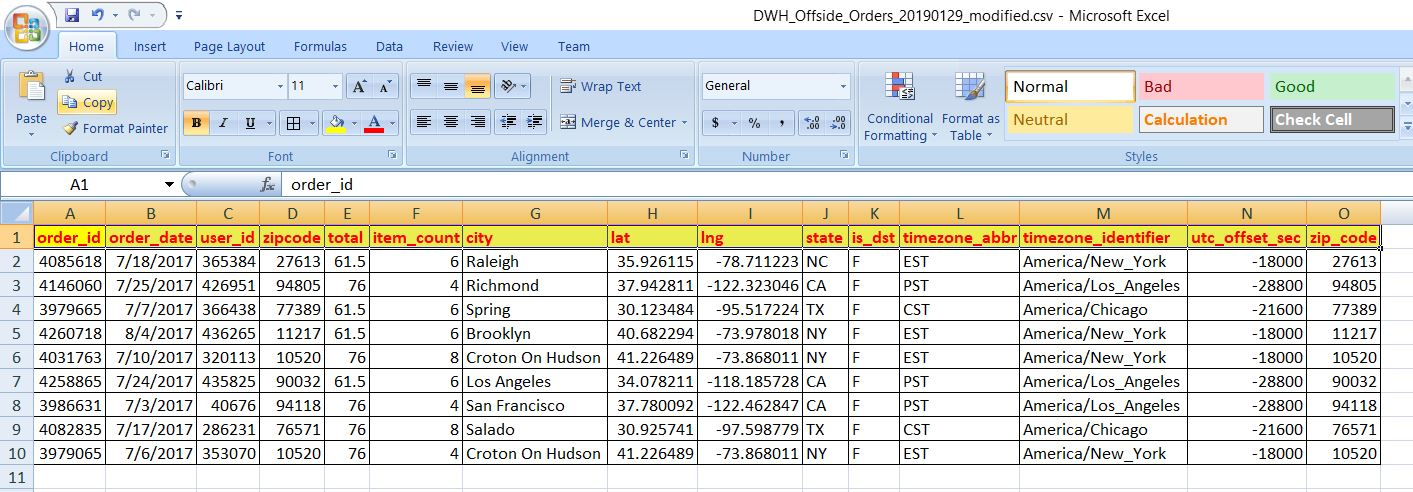From this screenshot, you can see how we have the desired intermediate data of City & State to proceed for the next level.

Let’s see how it runs –

Windows (64 bit):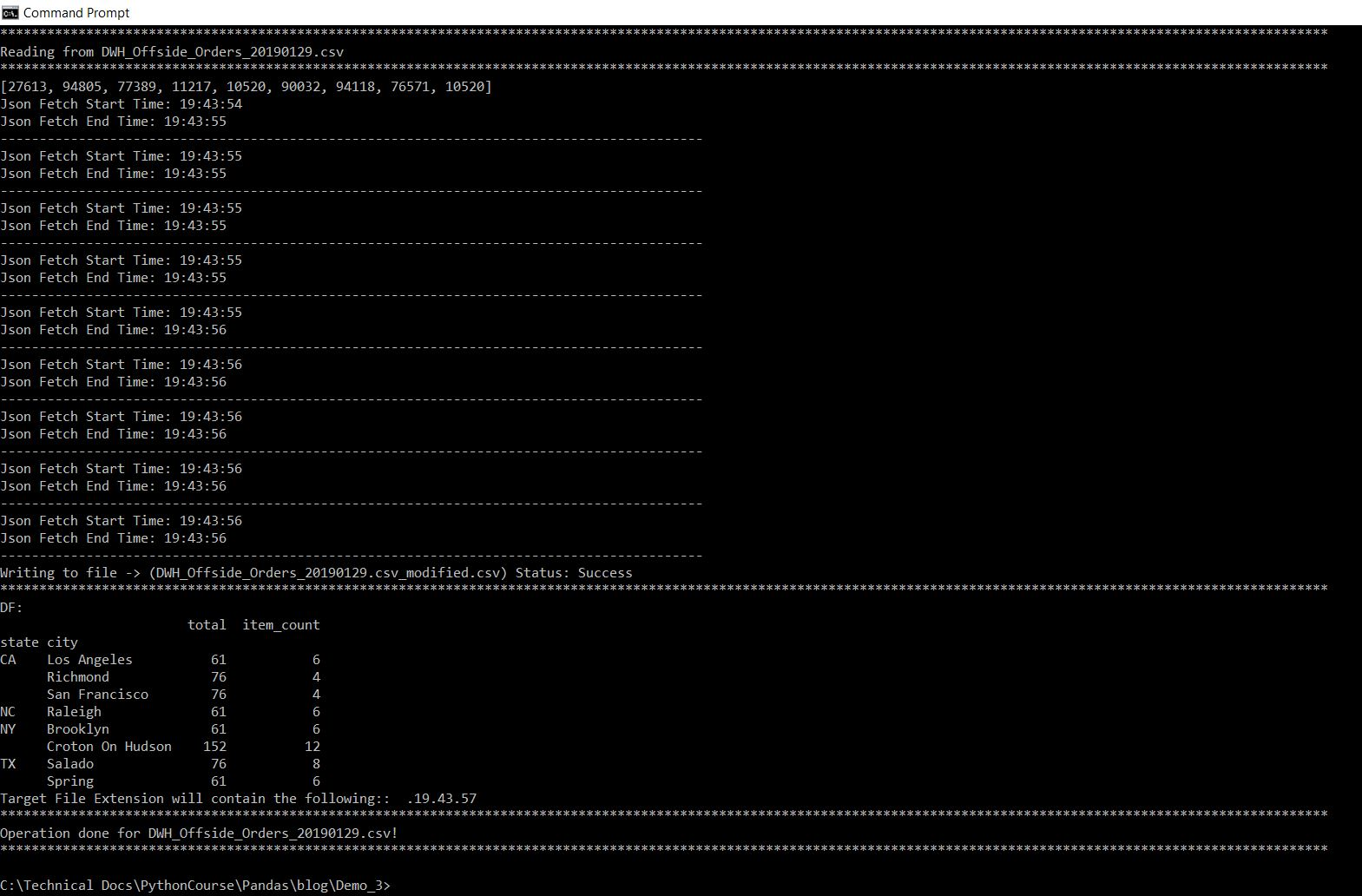Mac (32 bit):So, from the screenshot, we can see our desired output & you can calculate the aggregated value based on our sample provided in the previous screenshot.

Let’s check how the data directory looks like after run –

Windows: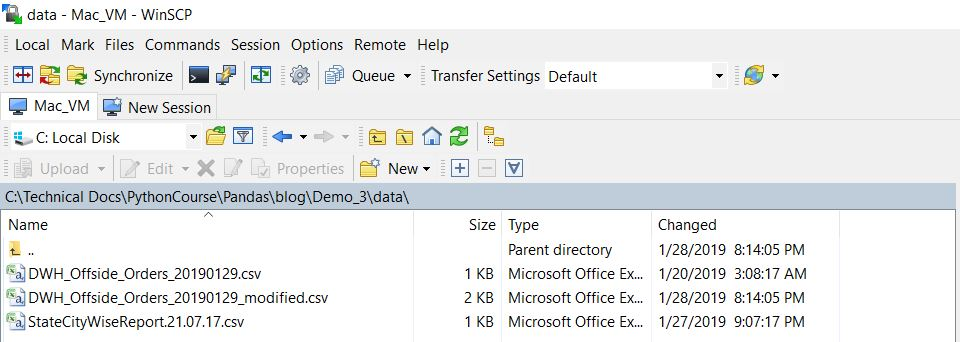MAC:So, finally, we’ve achieved our target.

I hope this will give you some more idea about more insights into the Python verse. Let me know – how do you think about this post.

Till then – Happy Avenging!

## Pandas & Numpy (Space Stone of Programming World)

Today, we’ll demonstrate the different application of Pandas. In this case, we’ll be exploring the possibilities of reading large CSV files & splitting it sets of smaller more manageable csv to read.

And, after creating it, another process will merge them together. This is especially very useful when you need transformation on a large volume of data without going for any kind of memory error. And, moreover, the developer has more control over failed cases & can resume the load without restarting it from the beginning of the files.

In this case, I’ll be using one more custom methods to create the csv file instead of directly using the to_csv method of pandas.

But, before that let’s prepare the virtual environment & proceed from there –

Windows 10 (64 bit):

##### Commands:

python -m venv –copies .env

.env\Scripts\activate.bat

##### Screenshot:Mac OS (64 bit):

##### Commands:

python -m venv env

source env/bin/activate

##### Screenshot:So, both the Windows & Mac version is 3.7 & we’re going to explore our task in the given section.

After creating this virtual environment, you need to install only pandas package for this task as shown below for both the Windows or Mac OS –

##### Windows: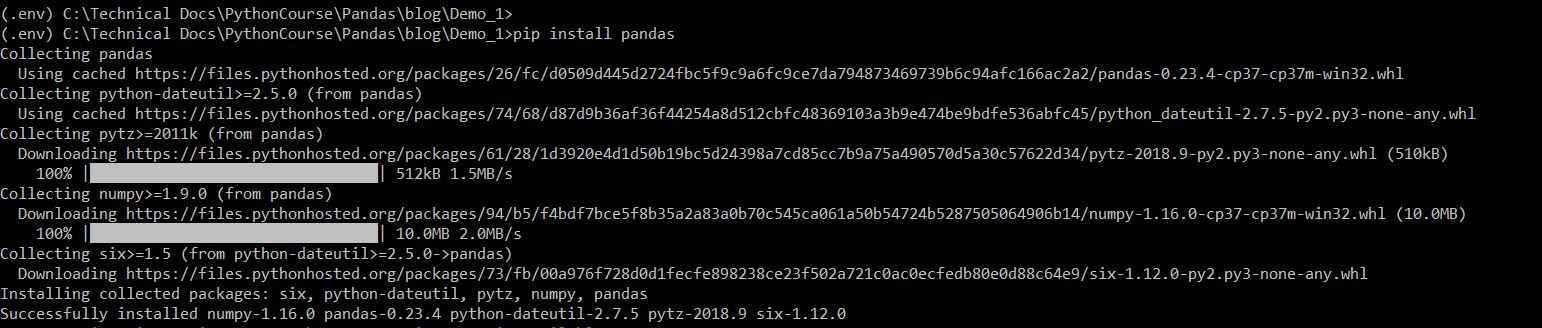##### Mac: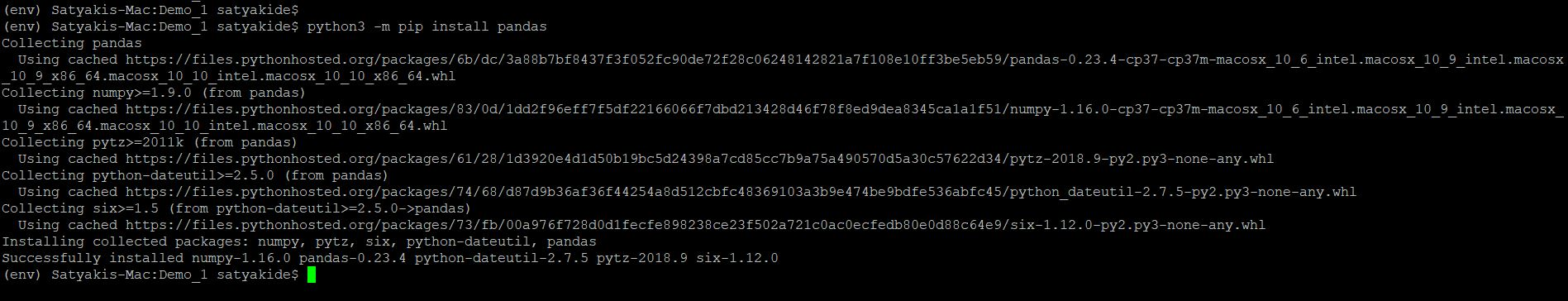Rests are the packages comes as default with the Python 3.7.

Please find the GUI screenshots from WinSCP software comparing both the directory structures (Mac & Windows) as given below –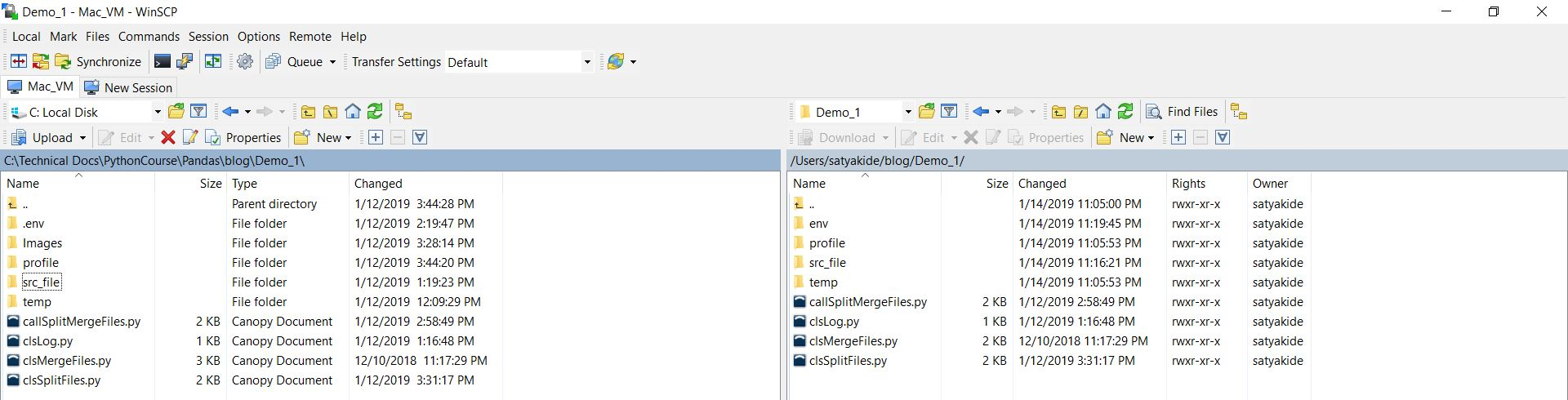From the above screenshot, you can see that our directory structure are not exactly identical before the blog directory. However, our program will take care of this difference.

Let’s check the scripts one-by-one,

1. clsL.py (This script will create the split csv files or final merge file after the corresponding process. However, this can be used as normal verbose debug logging as well. Hence, the name comes into the picture.)

 ``` 1 2 3 4 5 6 7 8 9 10 11 12 13 14 15 16 17 18 19 20 21 22 23 24 25 26 27 28 29 30 31 32 33 34 35 36 37 38 39 40``` ```############################################# #### Written By: Satyaki De #### ############################################# import pandas as p import os import platform as pl class clsL(object): def __init__(self): self.path = os.path.dirname(os.path.realpath(__file__)) def logr(self, Filename, Ind, df, subdir=None): try: x = p.DataFrame() x = df sd = subdir os_det = pl.system() if os_det == "Windows": if sd == None: fullFileName = self.path + "\\" + Filename else: fullFileName = self.path + "\\" + sd + "\\" + Filename else: if sd == None: fullFileName = self.path + "/" + Filename else: fullFileName = self.path + "/" + sd + "/" + Filename if Ind == 'Y': x.to_csv(fullFileName, index=False) return 0 except Exception as e: y = str(e) print(y) return 3 ```

From the above script, you can see that based on the Indicator, whose value can be either ‘Y’ or ‘N’. It will generate the csv file from the pandas data frame using to_csv method available in pandas.

Key snippet to notice –

self.path = os.path.dirname(os.path.realpath(__file__))

Here, the class is creating an instance & during that time it is initializing the value of the current path from where the application is triggering.

x = p.DataFrame()
x = df

The first line, declaring a pandas data frame variable. The second line assigns the value from the supplied method to that variable.

os_det = pl.system()

This will identify the operating system on which your application is running. Based on that, your path will be dynamically configured & passed. Hence, your application will be ready to handle multiple operating systems since beginning.

x.to_csv(fullFileName, index=False)

Finally, to_csv will generate the final csv file based on the supplied Indicator value. Also, notice that we’ve added one more parameter (index=False). By default, pandas create one extra column known as an index & maintain it’s operation based on that.As you can see that the first column is not coming from our source files. Rather, it is generated by the pandas package in python. Hence, we don’t want to capture that in our final file by mentioning (index=False) options.

2. clsSplitFl.py (This script will create the split csv files. This will bring chunk by chunk data into your memory & process the large files.)

 ``` 1 2 3 4 5 6 7 8 9 10 11 12 13 14 15 16 17 18 19 20 21 22 23 24 25 26 27 28 29 30 31 32 33 34 35 36 37 38 39 40 41 42 43 44 45 46 47 48 49 50 51 52 53 54 55 56 57 58 59 60 61 62 63 64 65 66 67``` ```############################################# #### Written By: Satyaki De #### ############################################# import os import pandas as p import clsLog as log import gc import csv class clsSplitFl(object): def __init__(self, srcFileName, path, subdir): self.srcFileName = srcFileName self.path = path self.subdir = subdir # Maximum Number of rows in CSV # in order to avoid Memory Error self.max_num_rows = 30000 self.networked_directory = 'src_file' self.Ind = 'Y' def split_files(self): try: src_dir = self.path subdir = self.subdir networked_directory = self.networked_directory # Initiate Logging Instances clog = log.clsLog() # Setting up values srcFileName = self.srcFileName First_part, Last_part = str(srcFileName).split(".") num_rows = self.max_num_rows dest_path = self.path remote_src_path = src_dir + networked_directory Ind = self.Ind interval = num_rows # Changing work directory location to source file # directory at remote server os.chdir(remote_src_path) src_fil_itr_no = 1 # Split logic here for df2 in p.read_csv(srcFileName, index_col=False, error_bad_lines=False, chunksize=interval): # Changing the target directory path os.chdir(dest_path) # Calling custom file generation method # to generate splitted files clog.logr(str(src_fil_itr_no) + '__' + First_part + '_' + '_splitted_.' + Last_part, Ind, df2, subdir) del [[df2]] gc.collect() src_fil_itr_no += 1 return 0 except Exception as e: x = str(e) print(x) return 1 ```

In this script, we’re splitting the file if that file has more than 30,000 records. And, based on that it will split a number of equal or fewer volume files.

Important lines to be noticed –

self.max_num_rows = 30000

As already explained, based on this the split files contain the maximum number of rows in each file.

First_part, Last_part = str(srcFileName).split(“.”)

This will split the source file name into the first part & second part i.e. one part contains only the file name & the other part contains only the extension dynamically.

As you can see, the chunk-by-chunk (mentioned as chunksize=interval) application will read lines from the large source csv. And, if it has any bad rows in the source files – it will skip them due to the following condition -> (error_bad_lines=False).

clog.logr(str(src_fil_itr_no) + ‘__’ + First_part + ‘_’ + ‘_splitted_.’ + Last_part, Ind, df2, subdir)

Dynamically generating split files in the specific subdirectory along with the modified name. So, these files won’t get overwritten – if you rerun it. Remember that the src_fil_itr_no will play an important role while merging them back to one as this is a number representing the current file’s split number.

del [[df2]]
gc.collect()

Once, you process that part – delete the data frame & deallocate the memory. So, that you won’t encounter any memory error or a similar issue.

And, the split file will look like this –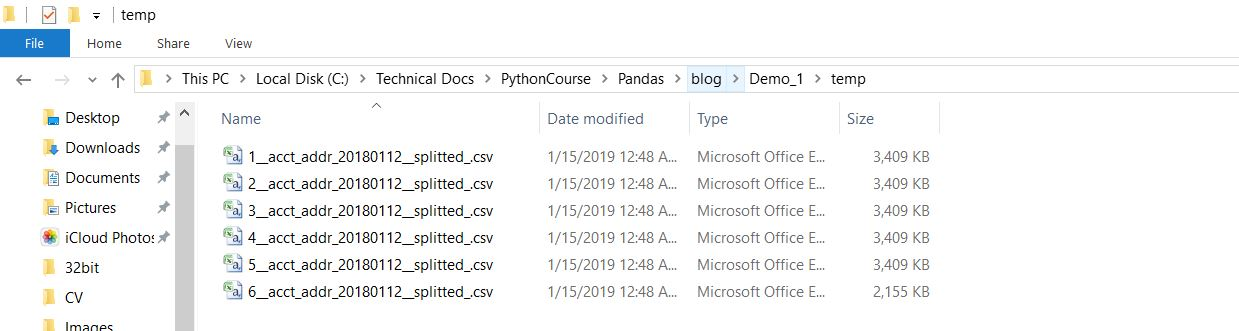3. clsMergeFl.py (This script will add together all the split csv files into one big csv file. This will bring chunk by chunk data into your memory & generates the large file.)

 ``` 1 2 3 4 5 6 7 8 9 10 11 12 13 14 15 16 17 18 19 20 21 22 23 24 25 26 27 28 29 30 31 32 33 34 35 36 37 38 39 40 41 42 43 44 45 46 47 48 49 50 51 52 53 54 55 56 57 58 59 60 61 62 63 64 65 66 67 68 69 70 71 72 73 74 75 76 77 78 79 80 81 82``` ```############################################# #### Written By: Satyaki De #### ############################################# import os import platform as pl import pandas as p import gc import clsLog as log import re class clsMergeFl(object): def __init__(self, srcFilename): self.srcFilename = srcFilename self.subdir = 'finished' self.Ind = 'Y' def merge_file(self): try: # Initiating Logging Instances clog = log.clsLog() df_W = p.DataFrame() df_M = p.DataFrame() f = {} subdir = self.subdir srcFilename = self.srcFilename Ind = self.Ind cnt = 0 os_det = pl.system() if os_det == "Windows": proc_dir = "\\temp\\" gen_dir = "\\process\\" else: proc_dir = "/temp/" gen_dir = "/process/" # Current Directory where application presents path = os.path.dirname(os.path.realpath(__file__)) + proc_dir print("Path: ", path) print("Source File Initial Name: ", srcFilename) for fname in os.listdir(path): if fname.__contains__(srcFilename) and fname.endswith('_splitted_.csv'): key = int(re.split('__', str(fname))) f[key] = str(fname) for k in sorted(f): print(k) print(f[k]) print("-"*30) df_W = p.read_csv(path+f[k], index_col=False) if cnt == 0: df_M = df_W else: d_frames = [df_M, df_W] df_M = p.concat(d_frames) cnt += 1 print("-"*30) print("Total Records in this Iteration: ", df_M.shape) FtgtFileName = fname.replace('_splitted_', '') first, FinalFileName = re.split("__", FtgtFileName) clog.logr(FinalFileName, Ind, df_M, gen_dir) del [[df_W], [df_M]] gc.collect() return 0 except Exception as e: x = str(e) print(x) return 1 ```

In this script, we’re merging smaller files into a large file. Following are the key snippet that we’ll explore –

```for fname in os.listdir(path):
if fname.__contains__(srcFilename) and fname.endswith('_splitted_.csv'):
key = int(re.split('__', str(fname)))
f[key] = str(fname)```

In this section, the application will check if in that specified path we’ve files whose extension ends with “_splitted_.csv” & their first name starts with the file name initial i.e. if you have a source file named – acct_addr_20180112.csv, then it will check the first name should start with the -> “acct_addr” & last part should contain “_splitted_.csv”. If it is available, then it will start the merge process by considering one by one file & merging them using pandas data frame (marked in purple color) as shown below –

```for k in sorted(f):
print(k)
print(f[k])
print("-"*30)

if cnt == 0:
df_M = df_W
else:
d_frames = [df_M, df_W]
df_M = p.concat(d_frames)

cnt += 1```

Note that, here f is a dictionary that contains filename in key, value pair. The first part of the split file contains the number.  That way, it would be easier for the merge to club them back to one large file without thinking of orders.

Here, also notice the special function concat provided by the pandas. In this step, applications are merging two data frames.

Finally, the main python script, from where we’ll call it –

4. callSplitMergeFl.py

 ``` 1 2 3 4 5 6 7 8 9 10 11 12 13 14 15 16 17 18 19 20 21 22 23 24 25 26 27 28 29 30 31 32 33 34 35 36 37 38 39 40 41 42 43 44 45 46 47 48 49 50 51 52 53 54 55 56 57 58 59 60 61 62``` ```############################################# #### Written By: Satyaki De #### ############################################# import clsSplitFl as t import clsMergeFl as cm import re import platform as pl import os def main(): print("Calling the custom Package for large file splitting..") os_det = pl.system() print("Running on :", os_det) ############################################################### ###### User Input based on Windows OS ######## ############################################################### srcF = str(input("Please enter the file name with extension:")) base_name = re.sub(r'[0-9]','', srcF) srcFileInit = base_name[:-5] if os_det == "Windows": subdir = "\\temp\\" path = os.path.dirname(os.path.realpath(__file__)) + "\\" else: subdir = "/temp/" path = os.path.dirname(os.path.realpath(__file__)) + '/' ############################################################### ###### End Of User Input ###### ############################################################### x = t.clsSplitFl(srcF, path, subdir) ret_val = x.split_files() if ret_val == 0: print("Splitting Successful!") else: print("Splitting Failure!") print("-"*30) print("Finally, Merging small splitted files to make the same big file!") y = cm.clsMergeFl(srcFileInit) ret_val1 = y.merge_file() if ret_val1 == 0: print("Merge Successful!") else: print("Merge Failure!") print("-"*30) if __name__ == "__main__": main() ```

Following are the key section that we can check –

import clsSplitFl as t
import clsMergeFl as cm

Like any other standard python package, we’re importing our own class into our main callable script.

```x = t.clsSplitFl(srcF, path, subdir)
ret_val = x.split_files()

Or,```
```y = cm.clsMergeFl(srcFileInit)
ret_val1 = y.merge_file()```

In this section, we’ve instantiated the class & then we’re calling its function. And, based on the return value – we’re printing the status of our application last run.

The final run of this application looks like ->

##### Windows: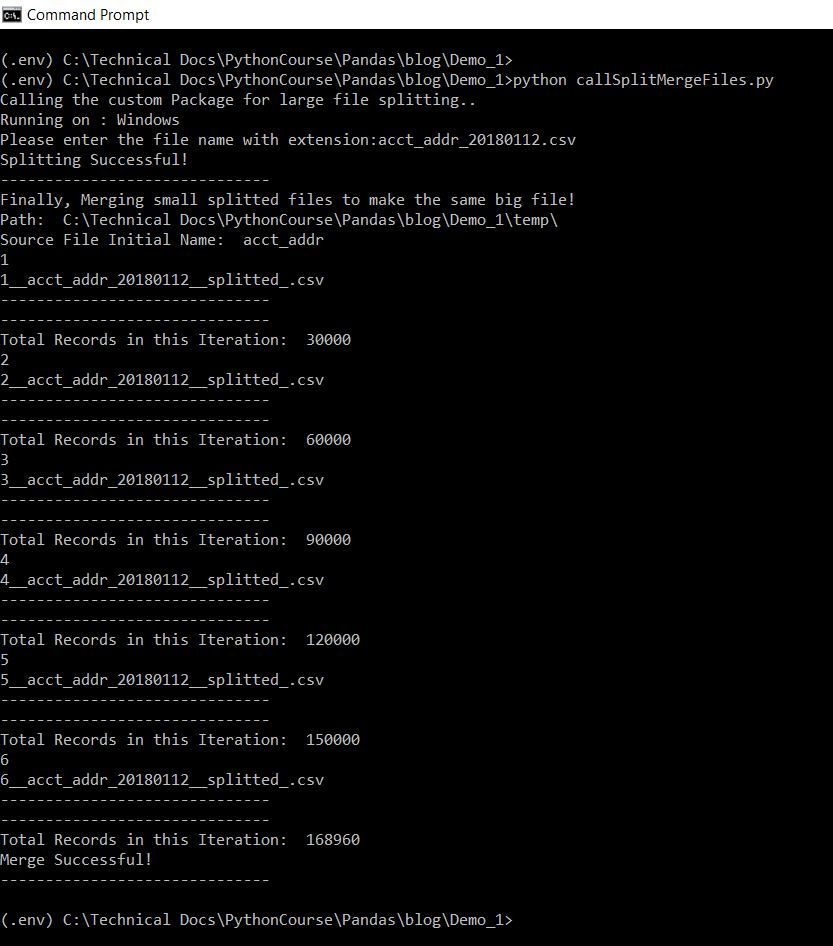##### Mac:And, the final file should look like this –

##### Windows:##### MAC:Left-hand side representing windows final processed/output file, whereas right-hand side representing MAC final processed/output file.

Hope, this will give you some idea about how we can use pandas in various cases apart from conventional data computing.

In this post, I skipped the exception part intentionally. I’ll post one bonus post once my series complete.

Let me know, what do you think.

Till then, Happy Avenging!

Satyaki De

## Python Verse – Universe of Avengers in Computer Language World!

The last couple of years, I’ve been working on various technologies. And, one of the interesting languages that I came across is Python. It is extremely flexible for developers to learn & rapidly develop with very few lines of code compared to the other languages. There are major versions of python that I worked with. Among them, python 2.7 & current python 3.7.1 are very popular to developers & my personal favorite.

There are many useful packages that are available to reduce the burden of the developers. Among them, packages like “pandas”, “numpy”, “json”, “AES”, “threading” etc. are extremely useful & one can do lot’s of work with it.

I personally prefer Ubuntu or Mac version of python. However, I’ve worked on Windows version as well or developed python based framework & application, which works in all the major operating systems. If you take care few things from the beginning, then you don’t have to make much more changes of your python application in order to work in all the major operating systems. 🙂

To me, Python Universe is nothing shorter than Marvel’s Universe of Avengers. In order to beat Supreme Villain Thanos (That Challenging & Complex Product with extremely tight timeline), you got to have 6 infinity stones to defeat him.

1. Space Stone ( Pandas & Numpy )
2. Reality Stone ( Json, SSL & Encryption/Decryption )
3. Power Stone ( Multi-Threading/Multi-Processing )
4. Mind Stone ( OS, Database, Directories & Files )
5. Soul Stone ( Logging & Exception )
6. Time Stone ( Cloud Interaction & Framework )

I’ll release a series of python based post in coming days, which might be useful for many peers or information seeker. Hopefully, this installment is a beginning & please follow my post. I hope, very soon you will get many such useful posts.

You get the latest version of Python from the official site given below –

Make sure you must install pip package along with python. I’m not going in details of how one should install python in either of Windows/Mac or Linux.

Just showing you how to install individual python packages.

Windows:

pip install pandas

Linux/Mac:

sudo python3.7 -m pip install pandas

From the second example, you can see that you can install packages to specific python version in case if you have multiple versions of python.

Note that: There might be slight variation based on different versions of Linux. Make sure you are using the correct syntax as per your flavor.

You can get plenty of good sites, where the detailed step-by-step process shared for each operating system.

Till then – Happy Avenging!